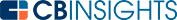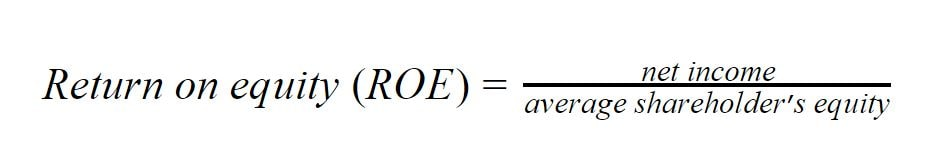# What is the ROE (Return on Equity) Equation?

The return on equity (ROE) equation is used to calculate a company’s returns on capital raised through equity.

Businesses need capital to sustain and grow their operations. Capital can be acquired in two ways: debt and equity. If a company wishes to calculate their returns on capital they raise, they can either use the ROE equation or the return on capital employed (ROCE) equation. While the ROE equation only focuses on returns raised through equity, ROCE considers the returns for both debt and equity.

## How to calculate return on equity (ROE)

Return on equity measures the efficiency of the company to generate returns on the capital raised through equity. Debt capital is not considered in this ratio to obtain the pure returns shareholders enjoy. It’s calculated as the ratio of net income and the average shareholder’s equity.Net income is the revenue after the cost of goods sold, operating expenses, interest paid, and taxes. This can be obtained from the profit and loss statement of the company.

In the return on equity equation, the average shareholder’s equity for the period is calculated. It is obtained by calculating the average of shareholder’s equity at the beginning of the year and at the end of the year.

Average shareholder's equity=(shareholder's equity at the beginning of the period + shareholder's equity at the end of the period)/2

Shareholders’ equity can be obtained from the company’s balance sheet. The financial year is typically the time period used for calculations. The values of shareholder equity should be taken from the balance sheet for the current year and the previous year. When trailing net income is used for calculations, the shareholder equity used in calculations should also reflect that.

Shareholders equity is in essence the difference between the company’s assets and debt. Return on equity can also be considered as the return on total assets.

## An example of return on equity

Company ABC has a net income of \$10M. The shareholder equity at the start of the financial year was \$80M and it grew to \$120M by the end of the financial year. You can calculate the company’s return on equity with the following steps.The return on equity for ABC for the financial year is 10%.

## Return on equity in the real world

Return on equity can be used to compare the capital efficiency of different investment opportunities. Different industries have different capital requirements, and it will be reflected in the return on equity for the industry. Airlines have a high need for capital compared to software companies. Airlines therefore generally have a lower return on equity than software companies.

Due to this, it’s best to compare the return on equity of peers in the same sector. This is more reliable than comparing the return on equity of companies operating in different sectors. A company with a return on equity greater than the average return on equity of its peers can be considered to be a higher quality company.

In addition to comparing different investment opportunities, return on equity can also be used to estimate the company’s future growth rate. Here, the assumption is that the amount of income retained after paying the dividends can generate a return that is equal to return on equity. The growth rate is estimated as the retention ratio and return on equity.

You may also like# www.cbinsights.com